• 邻接矩阵画有向图、无向图 由邻接矩阵画有向图的逻辑如下： 设邻接矩阵的行(列)数为N，矩阵中非零元素的个数为M，画布宽为W、高为H 1、有向图顶点数为N、有向边数为M，问题转化为：正N边形的所有顶点、以及顶点...
由邻接矩阵画有向图的逻辑如下：
设邻接矩阵的行(列)数为N，矩阵中非零元素的个数为M，画布宽为W、高为H
1、有向图顶点数为N、有向边数为M，问题转化为：画正N边形的所有顶点、以及顶点之间的M条有向线段
2、为方便作图，所有顶点位置相对于画布中心点(W/2, H/2)距离保持一致，该距离可设为一常量R(R>0)
3、两相邻顶点与画布中心点连线之间的夹角互为(2Π/N)
4、设顶点下标为i(0≤i<N)，顶点坐标可表示为(W/2*(1+cos(2Π/N*i+θ)), H/2*(1+sin(2Π/N*i+θ)))(θ为常量，0≤θ<2Π)
5、在画布上：根据顶点坐标画出N个像素点，再根据邻接矩阵非零元素画出这N个点之间的M条有向线段
注：常量R、θ可在有效范围内取任意值，以画出最适合的图形
画无向图的逻辑同上，只是M条有向线段变成(M/2)条无向线段

展开全文• 这里有一点一定要注意，因为为无向图，因此邻接矩阵一定要是关于对角线对称的，即Aij=Aji（且对角线上元素Aii=0），两点之间相互无向连接，有向图可以不为对称矩阵（有方向） 方法及函数： 1.推荐matlab一个图论很...
这里有一点一定要注意，因为为无向图，因此邻接矩阵一定要是关于对角线对称的，即Aij=Aji（且对角线上元素Aii=0），两点之间相互无向连接，有向图可以不为对称矩阵（有方向）

方法及函数：

1.推荐matlab一个图论很好用的包Graph and Network Algorithms，官网有函数介绍https://www.mathworks.com/help/matlab/graph-and-network-algorithms.html

这里搬一个知乎很不错评论的demo，仅供参考（参考链接文末一起放出）：

%% Matlab 无向图
% 生成数据,A和邻接矩阵的形势相似
A = magic(10);
A = dist(A)；%计算点之间的欧氏距离
index = 1:size(A,1);
names = {};
for ii = index
names{ii} = num2str(index(ii));
end
% 定义图像G
G = graph(A,names)
%% 绘制图像
figure
plot(G,'rs-','LineWidth',2,'MarkerSize',10)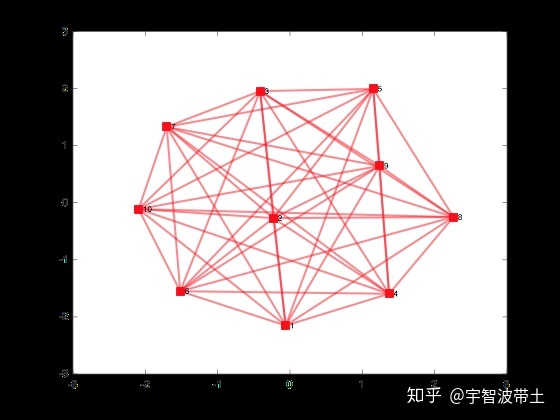还可以改变各个节点之间的绘图方式

%% 绘制图像 定义图像的图层为圆形
figure
plot(G,'ro-','Layout','circle','LineWidth',2,'MarkerSize',10)
<img src="https://pic1.zhimg.com/50/v2-96c490f5c4126fa150e5bc56688bc6db_hd.jpg" data-caption="" data-size="normal" data-rawwidth="560" data-rawheight="420" data-default-watermark-src="https://pic3.zhimg.com/50/v2-e4b7648f26b717dba1f56b58662e9698_hd.jpg" class="origin_image zh-lightbox-thumb" width="560" data-original="https://pic1.zhimg.com/v2-96c490f5c4126fa150e5bc56688bc6db_r.jpg"/>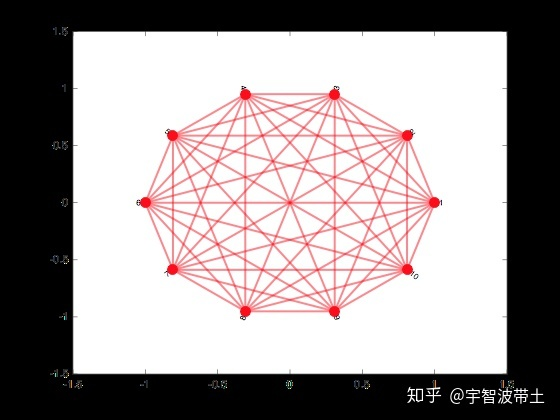如果每个节点是有坐标的，也可以指定节点在空间中的位置

%% 指定每个点的坐标
x = randperm(10)
y = randperm(20,10)
figure
p = plot(G,'ro-','LineWidth',2,'MarkerSize',10)
p.XData = x
P.YData = y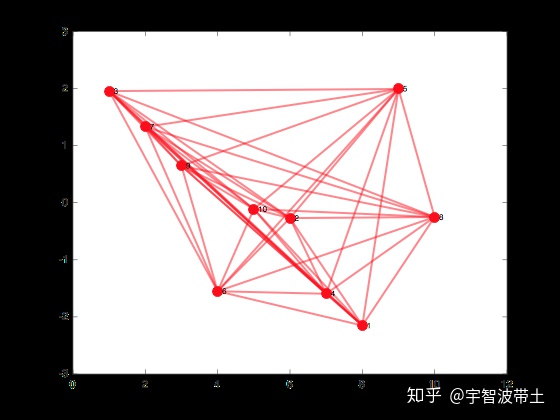2.使用gplot（）

gplot(A, xy):
A表示邻接矩阵，xy表示每个点的位置

如下定义一个函数 netplot，将每个点均匀地放置在单位圆上以画出邻接矩阵：

function netplot(G)
n = size(G, 1);
[x y] = pol2cart((0:n-1)*2*pi/n, 1);
gplot(G, [x' y'], '-o');
end

[X,Y] = POL2CART(TH,R)将极坐标(角度TH和半径R)存储的数据中相应的元素转换到笛卡儿坐标X,Y。数组TH和R必须大小相同(或者二者皆可标量)，TH必须是弧度值。

[X,Y,Z] = POL2CART(TH,R,Z)将圆柱坐标(角度TH、半径R和高度Z)存储的数据中相应的元素转换到笛卡儿坐标X,Y，Z。数组TH、R和Z必须大小相同(或者其中任何一个皆可标量)，TH必须是弧度值。

调用如示：

a = [0 1 1 0; 1 0 1 0; 1 0 0 1; 0 0 1 0];
netplot(a);

输出如图所示：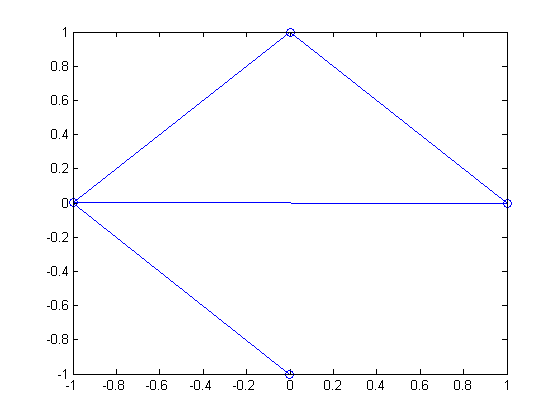参考网址：

https://www.zhihu.com/question/275337007

https://zhidao.baidu.com/question/680827699342938012.html

展开全文机器学习
• 有向图不对称邻接矩阵变无向图对称邻接矩阵 一个简单图实例 import numpy as np # 使用numpy编写的上述有向图邻接矩阵如下： # 不对称邻接矩阵 A = np.matrix([ [0,1,0,0], [0,0,1,1], [0,1,0,0], [1,0,1,0]...
有向图不对称邻接矩阵变无向图对称邻接矩阵
一个简单图实例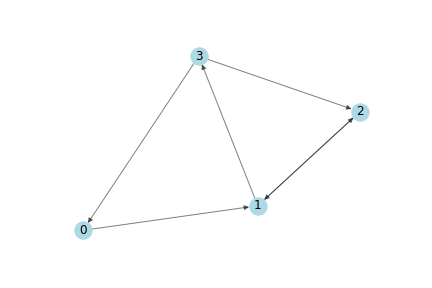import numpy as np

# 使用numpy编写的上述有向图的邻接矩阵如下：
# 不对称邻接矩阵
A = np.matrix([
[0,1,0,0],
[0,0,1,1],
[0,1,0,0],
[1,0,1,0]],
dtype=float
)

A.T

matrix([[0., 0., 0., 1.],
[1., 0., 1., 0.],
[0., 1., 0., 1.],
[0., 1., 0., 0.]])

a = A.T > A
a=a.astype(float)
a

matrix([[0., 0., 0., 1.],
[1., 0., 0., 0.],
[0., 0., 0., 1.],
[0., 1., 0., 0.]])

np.multiply(A.T,a)

matrix([[0., 0., 0., 1.],
[1., 0., 0., 0.],
[0., 0., 0., 1.],
[0., 1., 0., 0.]])

# 变为对称邻接矩阵
A = A + np.multiply(A.T,a) - np.multiply(A,a)
A

matrix([[0., 1., 0., 1.],
[1., 0., 1., 1.],
[0., 1., 0., 1.],
[1., 1., 1., 0.]])



展开全文numpy
• /* 邻接矩阵、构造有向图 */ #include #include const int maxv = 20; //INF定义为无穷大，表示不连通 const int INF = 9999; bool visited[maxv];/* MGraph类（邻接矩阵构建图类）*/ class MGraph { private: ...
/* 邻接矩阵、构造有向图 */
#include <cstdio>
#include <cstdlib>
const int maxv = 20;
//INF定义为无穷大，表示不连通
const int INF = 9999;
bool visited[maxv];

/* MGraph类（邻接矩阵构建图类）*/
class MGraph
{
private:
typedef struct
{
int edges[maxv][maxv];
int n,e;
}Graph;//定义图类型

Graph *g;//定义图类型变量g

public:
MGraph()//构造函数内，初始化成员g
{
g = (Graph*)malloc(sizeof(Graph));
}
/* 构建图（邻接矩阵） */
void create_MGraph()
{
printf("输入顶点数,边数(n e): ");
scanf("%d%d",&g->n,&g->e);
int i,j,w;
for(int i = 0; i < g->n; i++)
{
for(int j = 0; j < g->n; j++)
{
g->edges[i][j] = INF;
}
}
printf("输入边及权(i j weight):\n");
for(int count = 0; count < g->e; count++)
{
scanf("%d%d%d",&i,&j,&w);
g->edges[i][j] = w;
}
}
/* 打印图（邻接矩阵） */
void print_MGraph()
{
for(int i = 0; i < g->n; i++)
{
for(int j = 0; j < g->n; j++)
{
printf(" %4d ",g->edges[i][j]);
}
printf("\n");
}
}
/* 深度优先遍历 */
void dfs(int v)
{
printf("V%d ",v);
visited[v] = 1;
int w;
for(w = 0; w < g->n; w++)
if(g->edges[v][w]!=0 && visited[w] == 0)
dfs(w);
}
/* 广度优先遍历 */
void bfs(int v)
{
int qu[maxv];
int front = 0,rear = 0;

printf("V%d ",v);
visited[v] = 1;
rear = (rear+1) % maxv;
qu[rear] = v;
int w;
while(front != rear)
{
front = (front+1) % maxv;
w = qu[front];
for(int i = 0; i < g->n; i++)
{
if(g->edges[w][i] != 0 && visited[i] == 0)
{
printf("V%d ",i);
visited[i] = 1;
rear = (rear+1) % maxv;
qu[rear] = i;
}
}
}
}
/* 输出任意顶点V0，到其他顶点的最短路径 */
void Dispath(int dist[],int path[],int S[],int v)
{
int k,d;
for(int i = 0; i < g->n; i++)
if(S[i] == 1 && i != v)
{
printf("V%d->V%d的路径长度为: %d\n",v,i,dist[i]);
k = path[i];
if(k == -1)
printf("无路径\n");
}
}
/* Dijkstra算法，求最短路径 */
void Dijkstra(int v)
{
int dist[maxv],path[maxv];
int S[maxv];
int mindist,u;
for(int i = 0; i < g->n; i++)
{
dist[i] = g->edges[v][i];
S[i] = 0;
if(g->edges[v][i] < INF)
path[i] = v;
else
path[i] = -1;
}
S[v] = 1,path[v] = 0;
for(int i = 0; i < g->n; i++)
{
mindist = INF;
for(int j = 0; j < g->n; j++)
{
if(S[j] == 0 && dist[j] < mindist)
{
u = j;
mindist = dist[j];
}
}
S[u] = 1;
for(int j = 0; j < g->n; j++)
{
if(S[j] == 0)
{
if(g->edges[u][j] < INF && dist[u] + g->edges[u][j] < dist[j])
{
dist[j] = dist[u] + g->edges[u][j];
path[j] = u;
}
}
}
}
Dispath(dist,path,S,v);
}
/* g->n为MGraph类私有成员
只能通过函数访问，获得图的边数n */
int MGraph_n()
{
return g->n;
}
};

int main()
{
MGraph mgraph;//MGraph类，实例化对象mgarph
//freopen("data.txt","r",stdin);
printf("create MGraph（邻接矩阵类）\n");
mgraph.create_MGraph();
printf("---------------------\n");
printf("print MGraph（邻接矩阵类）\n");
mgraph.print_MGraph();
printf("---------------------\n");
/* 深度优先遍历 */
for(int i = 0; i < mgraph.MGraph_n(); i++)
visited[i] = 0;
printf("dfs: ");
mgraph.dfs(0);
printf("\n");
/* 广度优先遍历 */
for(int i = 0; i < mgraph.MGraph_n(); i++)
visited[i] = 0;
printf("bfs: ");
mgraph.bfs(0);
printf("\n");
printf("---------------------\n");
/* 输出任意顶点V0，到其他顶点的最短路径 */
mgraph.Dijkstra(0);

system("Pause");
return 0;
}
/*
测试用例:
//（第一组）
4 4
0 1 9
1 2 8
0 2 7
3 2 6

//（第二组）
5 7
0 1 9
1 2 8
2 3 7
3 4 6
0 2 5
0 3 4
0 4 3
*/
展开全文• 一、邻接矩阵有向图的介绍 邻接矩阵有向图是指通过邻接矩阵表示的有向图。 待补充； 上面的图G2包含了"A,B,C,D,E,F,G"共7个顶点，而且包含了"<A,B>,<B,C>,<B,E>,<B,F>,<C,E&...
• 邻接矩阵表示一个n个顶点的有向图时，所需空间为n*n。输入邻接矩阵和查询一遍口需要n*n的时间。当图的边数远小于n*n时，该方法就会很浪费时间和空间，而用邻接表来表示图会更有效。 #include #instruct 数据结构 存储 二维数组
• Python语言，用邻接矩阵实现图 通过二维数组建立无向图 通过二维数组建立有向图 通过边建立有向图 为方便查看，通过NetworkX显示图。
• 简单方法判断邻接矩阵有向图是否有环求解思路实现代码如有不同见解，欢迎讨论 求解思路 1.统计所有节点的出度及其前序节点，初始化一个空的访问集合； 2.将出度为零的节点放入访问集合，并将其前序节点的出度数减1...java leetcode
• 邻接矩阵存储有向图，并输出各定点的出入和入度 输入描述： 输入文件中包含多个测试数据，每个测试数据描述了一个无权有向图。每个测试数据的第一行为两个正整数n和m，1<=n<=100,1<=m<=500，分别...
• 1构成一个环，所以返回1表示环 输入：[[0,1,0],[0,0,1],[0,0,0]] 输出：0 解释：1->2->3，这是一条拓扑路径，所以返回0表示没有环 #include<iostream> #include<vector> #i...
• 1.DigraphConnectivity import java.util.ArrayList... * 构造邻接矩阵，并用邻接矩阵解决有向图的连通性问题  * 问题描述：给定两颗钻石的编号g1,g2，编号从1开始，同时给定关系数组vector,其中元素为一些二元组，
• 邻接矩阵存储有向图，并输出各顶点的出度和入度。 输入 输入描述： 输入文件中包含多个测试数据，每个测试数据描述了一个有向图。每个测试数据的第一行为一个正整数n (1≤n≤100)，表示该有向图的顶点数目，顶点...
• #include #include #include #define MAXSIZE 100 #define MaxInt 32767 //表示最大值，即正无穷大 #define ... } } //输出邻接矩阵 void PrintAM(AMGraph &G){ printf("\n输出邻接矩阵:\n"); for(int i=0;i
• 邻接矩阵存储有向图 【输入描述】  输入文件包含多组测试数据，每组测试数据描述了一个无权有向图。每组测试数据第一行为两个正整数n和m，1 【输出描述】：  对输入文件的每个有向图，输出两行：第一行为n个正...
• 网上查了很多资料，发现主要是使用邻接表来实现图，并进行遍历的。而采用邻接矩阵的就非常少...不想看的可以直接下载：python 邻接矩阵三种方法实现有向图、无向图，并绘图显示不废话。上代码首先图类 class Graph_Matrpython 二维数组 显示
• 利用Gephi软件根据邻接矩阵构建网络拓扑1.导入excel数据2.输出节点和边3.导入节点和边4.调整网络拓扑 下面讲述了怎么利用excel表中的邻接矩阵构建网络拓扑，对于大的邻接矩阵特别方便 1.导入excel数据 下为...excel
• 邻接矩阵表示赋权无向图时，将一个赋权无向图G当作一个赋权有向图来处理，将G的每一条边(i,j)，用两条有向边(i,j)和(j,i)来代替。因此，加入边权为w的有向边(i,j）时，还应同时加入边权为w的有向边(j,i)； 删除边...存储 数据结构 二维数组 malloc struct
• 邻接矩阵有向图的介绍邻接矩阵有向图是指通过邻接矩阵表示的有向图。上面的图G2包含了”A,B,C,D,E,F,G”共7个顶点，而且包含了”邻接矩阵有向图的代码说明1. 基本定义public class MatrixDG { private char[] mVexs;...
• 邻接矩阵实现无权无向图、带权无向图 java 1.图的接口 import java.util.List; public interface Graph { public int getSize(); //返回图中的顶点数 public List getVertices(); //返回图形中的顶点 public V ge...java
• 给定带权有向图G=(V,E)，对于任意顶点Vi到Vj(i!=j),求Vi到Vj的最短路径。 基本思想： 假设从vi到vj的弧(若从vi到vj的弧不存在，则将其弧的权值看成无穷大)是最短路径，然后进行n次试探。首先比较vi，vj和vi，v0，vj...c++ Floyd算法 最短路径
• //数值类型 整型//创建有向图邻接矩阵//把邻接矩阵转换成临界表//把值大于50的顶点保存在单链表中//输出邻接矩阵，临界表，大于50的结点#include&lt;stdio.h&gt;#include&lt;malloc.h&gt;#define ...单链表
• /*********************邻接矩阵实现无向图******************/ class MatrixUDG { private: char mVexs[MAX];//顶点集合 int mVexBum;//顶点数 int mEdgNum;//边数 int mMatrix[MAX][MAX];//邻接矩阵 char ...c语言
• 上一个文章是写的无向图和邻接链表的广度搜索，深度搜索就用矩阵有向图了。 矩阵处理起来还是会比链表简单一些。 先分析数据结构： 1.储存所有结点的集合用到了一个单向循环链表，为什么要用循环链表呢，因为在...深度优先搜索
• Python求解：利用邻接矩阵判断有向图的连通性 WoW，让我们先来看看我们要解决的小问题: 将任意一个有向图G采用矩阵输入，图形化输出图G，利用可达矩阵判定图G是否连通： （PS：判断图的连通性至少可以有.并...python numpy
• ## 邻接矩阵--无向图

千次阅读 2017-04-24 18:05:54
邻接矩阵存储方式是用俩个数组来表示的。一个一维数组储存中顶点信息，一个二维数组（称为邻接矩阵）存储中的边和弧的信息。 了矩阵的好处： 1、我们要判定任意二个顶点是否边就容易了； 2、我们...
• ## 邻接矩阵有向图

千次阅读 2018-08-14 22:45:15
和无向图的非常相似，只是反向不记录为1而已 #include &lt;stdio.h&gt; #include &lt;stdlib.h&gt; #include &lt;malloc.h&gt; #include &lt;string.h&gt; #define MAX 100 #...
• 多组数据，每组数据第一行两个整数n、m,(0  Input 分别输出从标号为1点开始深度和广度优先搜索的序列，每个数之后一个空格。 每组数组分别占一行。  Output 按存储顺序的先后，输出各连通分量...bfs dfs
• Matlab中根据邻接矩阵做图 function tu_plot(rel,control) ...%第二个输入为控制量，0表示无向图，1表示有向图。默认值为0 r_size=size(rel); if nargin<2 control=0; end if r_size(1)~=r_size(2) di...机器学习...